Wiki Home Miner USDT ERC20 address

For the ERC20 address, you need to create a binance account. Follow the following steps: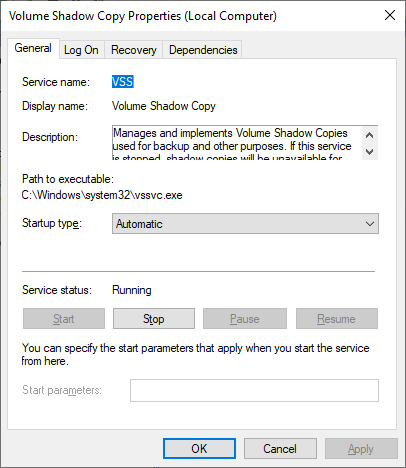Figure 1

1. Enter the code sent to your email account (Figure 2).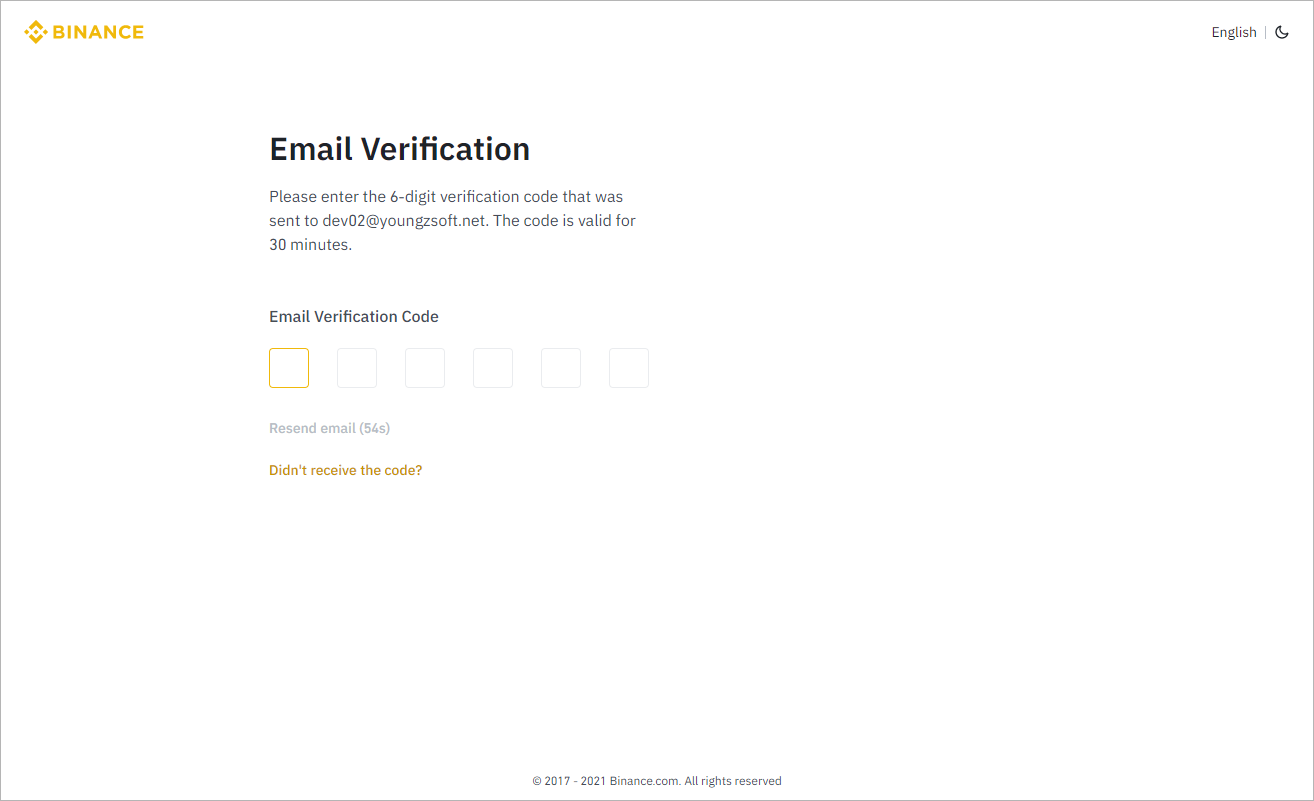Figure 2

1. Then go to the dashboard (Figure 3).Figure 3

1. Click the “Wallet” dropdown and select “Fiat and Spot” (Figure 4).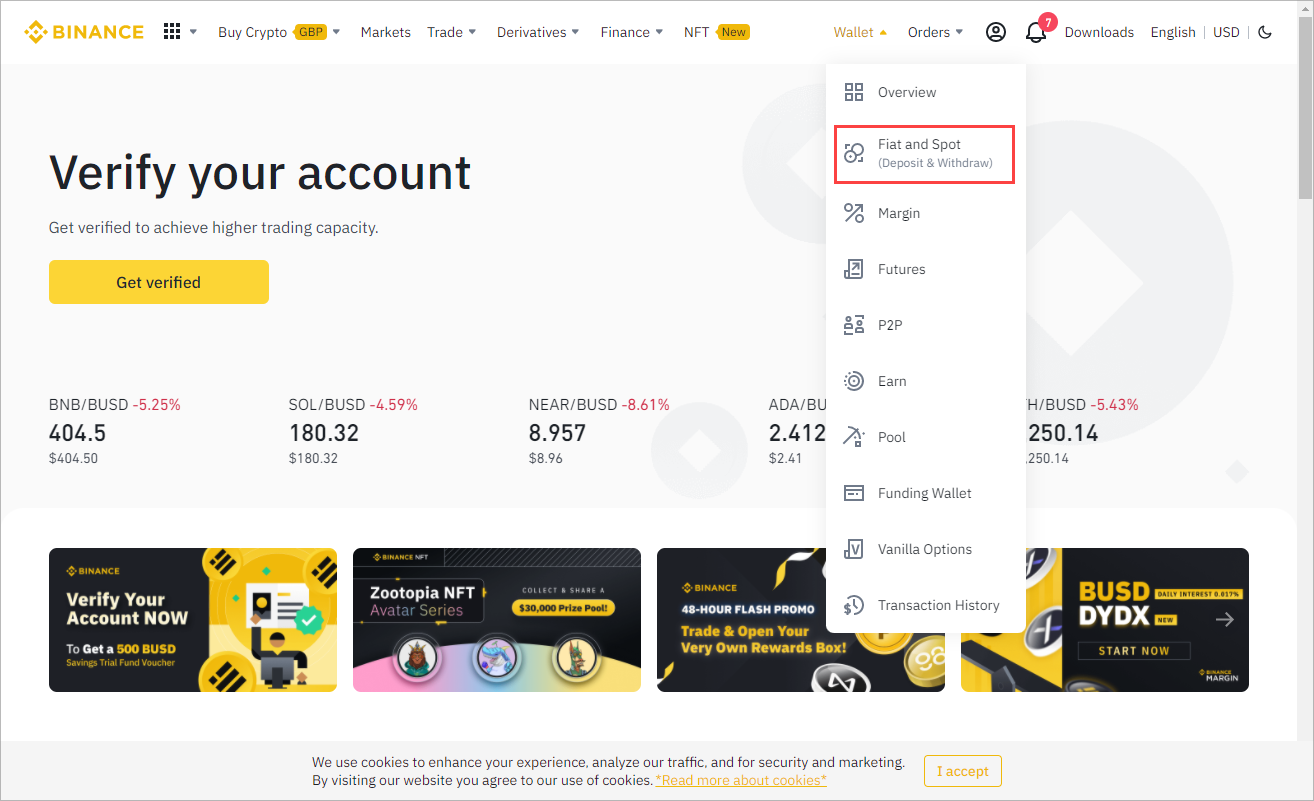Figure 4

1. Select usdt and click the deposit button (Figure 5).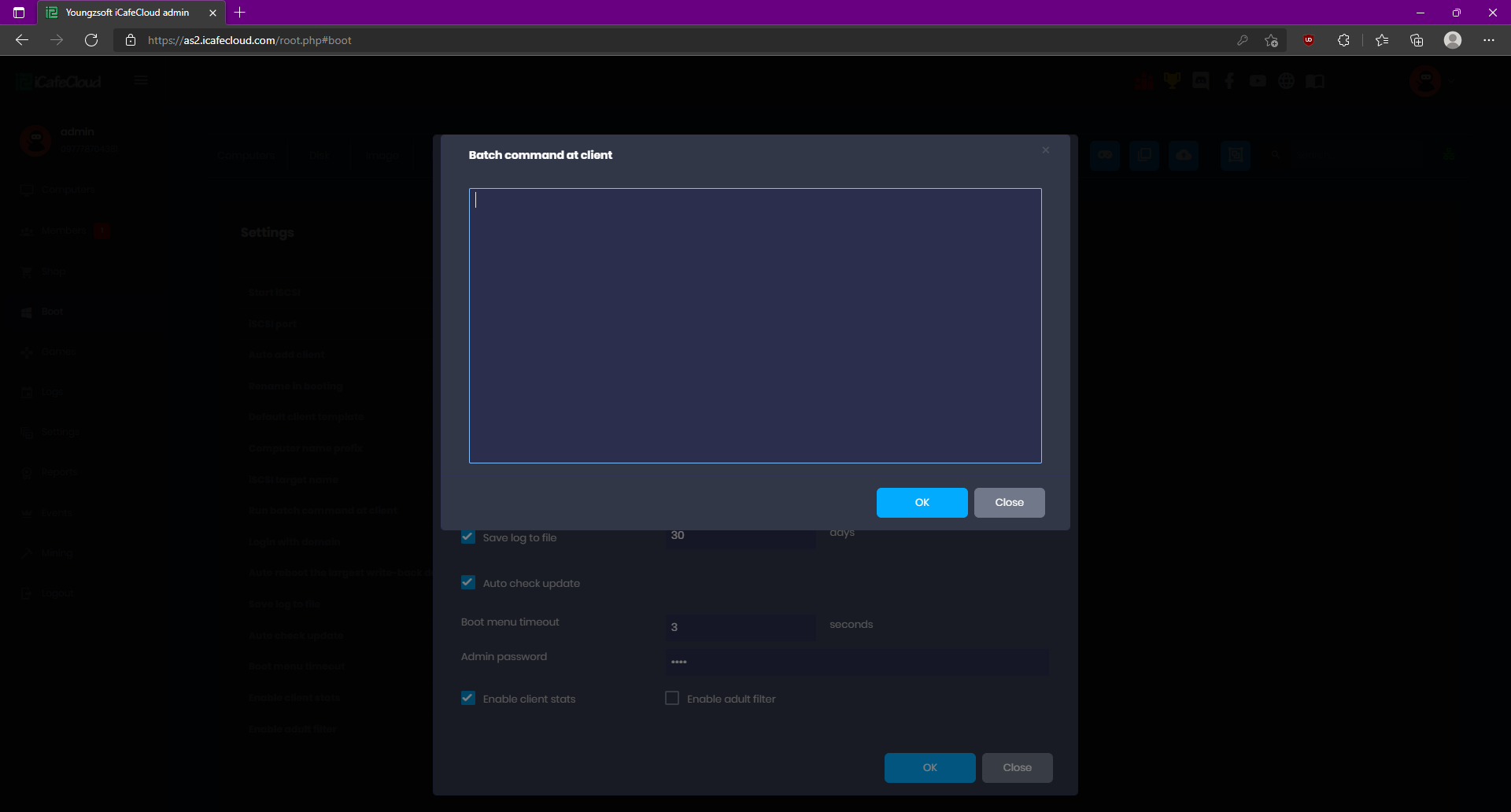Figure 5

1. Select “ETH” in the network field .Then the ERC20 address will be displayed which you can copy and paste(Figure 6).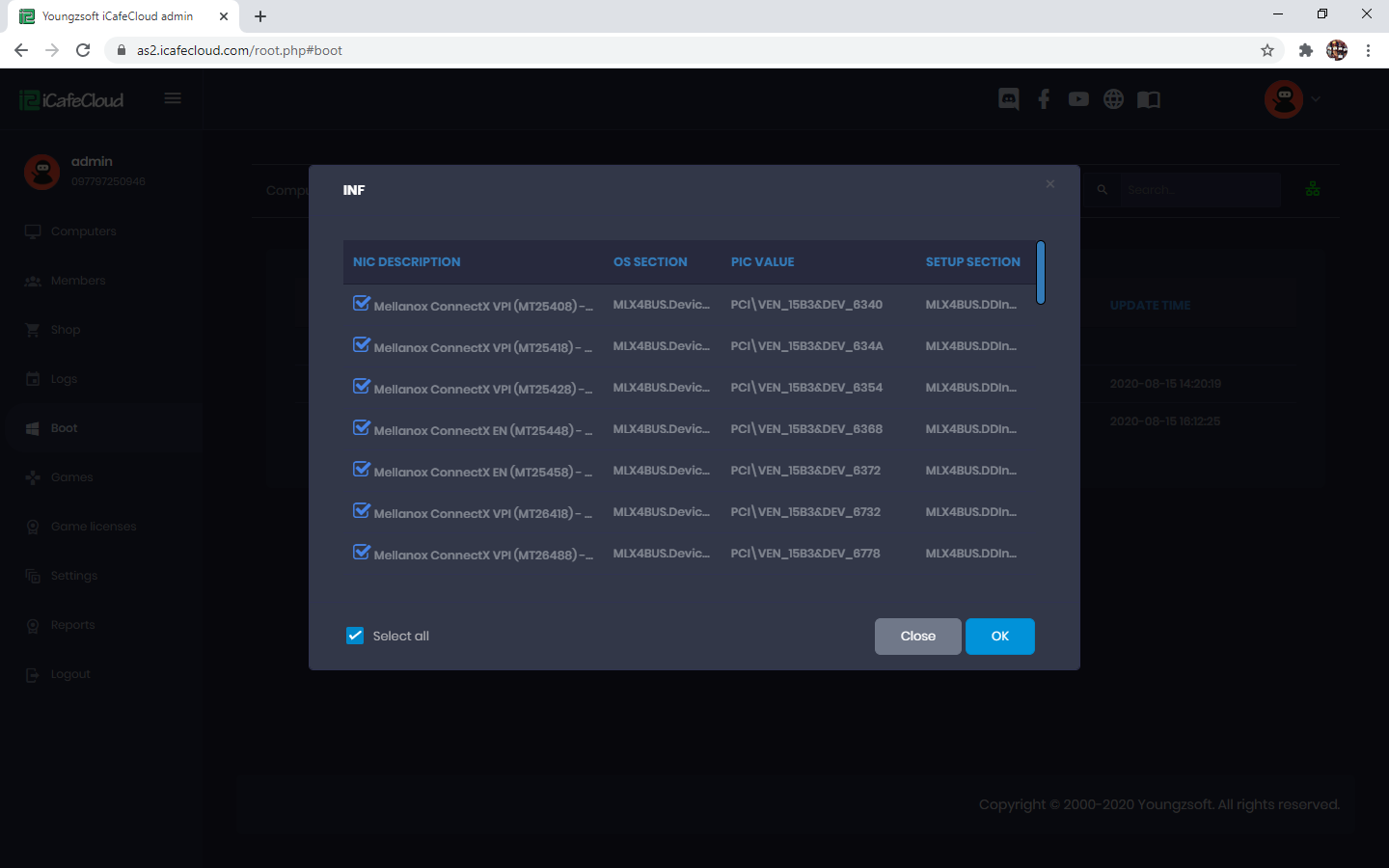Figure